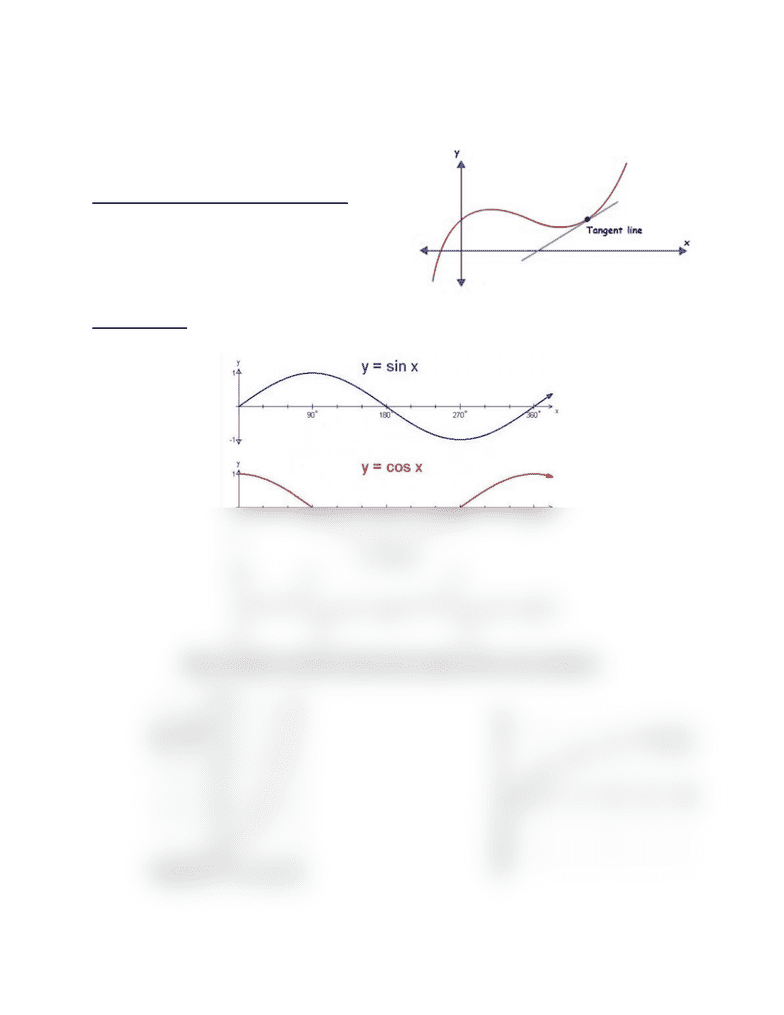# MATH 2A Lecture Notes - Lecture 5: Asymptote

66 views2 pages
School
Department
Course
ProfessorMath 2A Lecture 5- Comparing Limits
Limit f(x) = L
x-->a
“The limit of f(x) as x approaches a is L.”
The tangent line: y-y0= f’(x0)(x-x0)
Picture: https://calculator.tutorvista.com/tangent-line-calculator.html
Basic Graphs:
https://owlcation.com/stem/Trigonometry-graphs-of-sin-x-cos-x-and-tan-x
Unlock document

This preview shows half of the first page of the document.
Unlock all 2 pages and 3 million more documents.

## Document Summary

The limit of f(x) as x approaches a is l. Y = 2x graph: https://people. richland. edu/james/lecture/m116/logs/2x. gif y=log 2 x graph: https://upload. wikimedia. org/wikipedia/commons/thumb/1/17/binary_logarithm_plot_with_ticks. svg/300px-binary_logarithm_plot_wit h_ticks. svg. png. This means that the left-handed limit less than a a is equal to l if we can get x to be greater than a. As f(x) approaches a is equal to l if we can get x to be. This is also true the other way around. Lim f(x) = lim f(x) = - x-->a x-->a. This means that the values of f(x) can be made as large as we"d like a, but not equal to a. The values of f(x) can be made as small as we"d like . A vertical line, called x=a will be considered a vertical asymptote of y= f(x) if at least one of these statements is true: By taking x to be close to a, but not equal to a. These are straight lines of the equation that the function f(x) approaches,

## Get access

\$8 USD/m\$10 USD/m
Billed \$96 USD annuallyHomework Help
Study Guides
Textbook Solutions
Class Notes
Textbook Notes
Booster Class